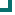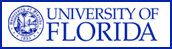Enterococcus faecium (NORTHEAST Data) 2004 2005 2006 2007 2008 2009 2010 ampicillin 10.3%n=677 9.1%n=615 10%n=1350 13.6%n=1113 11.3%n=1313 11.4%n=333 vancomycin 41.6%n=1047 43%n=987 29.9%n=1668 30.8%n=1359 30%n=1469 29.8%n=416 quinupristin/dalfopristin 65.2%n=89 67.6%n=34 87.4%n=127 95.8%n=48 100%n=58 100%n=45 nitrofurantoin 56.9%n=561 41%n=444 24.2%n=1207 9.6%n=934 11.7%n=966 5.4%n=204 tetracycline 38.3%n=630 54.3%n=479 56.3%n=1033 59.3%n=848 62.6%n=1146 52.5%n=204 gentamicin 73.1%n=443 77.1%n=48 84%n=200 80%n=75 0%n=140 amikacin 9.1%n=11 chloramphenicol 95.8%n=331 100%n=58 98.3%n=705 100%n=37 100%n=33 rifampim 2.6%n=38 0%n=22 Totals 46.7%n=3778 39%n=2665 39.2%n=6328 29.6%n=4414 29.3%n=5147 26.9%n=1213

This data is accurate as of: 11/22/2016 11:53:33 AM

Drugs with less than 10 isolates are removed from the chart and from the graph. The following drugs had some data removed: quinupristin/dalfopristin, gentamicin, chloramphenicol, rifampim, amikacin.ARMProgram.com is a project run by the University of Florida. Copyright 2011. All Rights Reserved. Site by JMarc Technologies, LLC